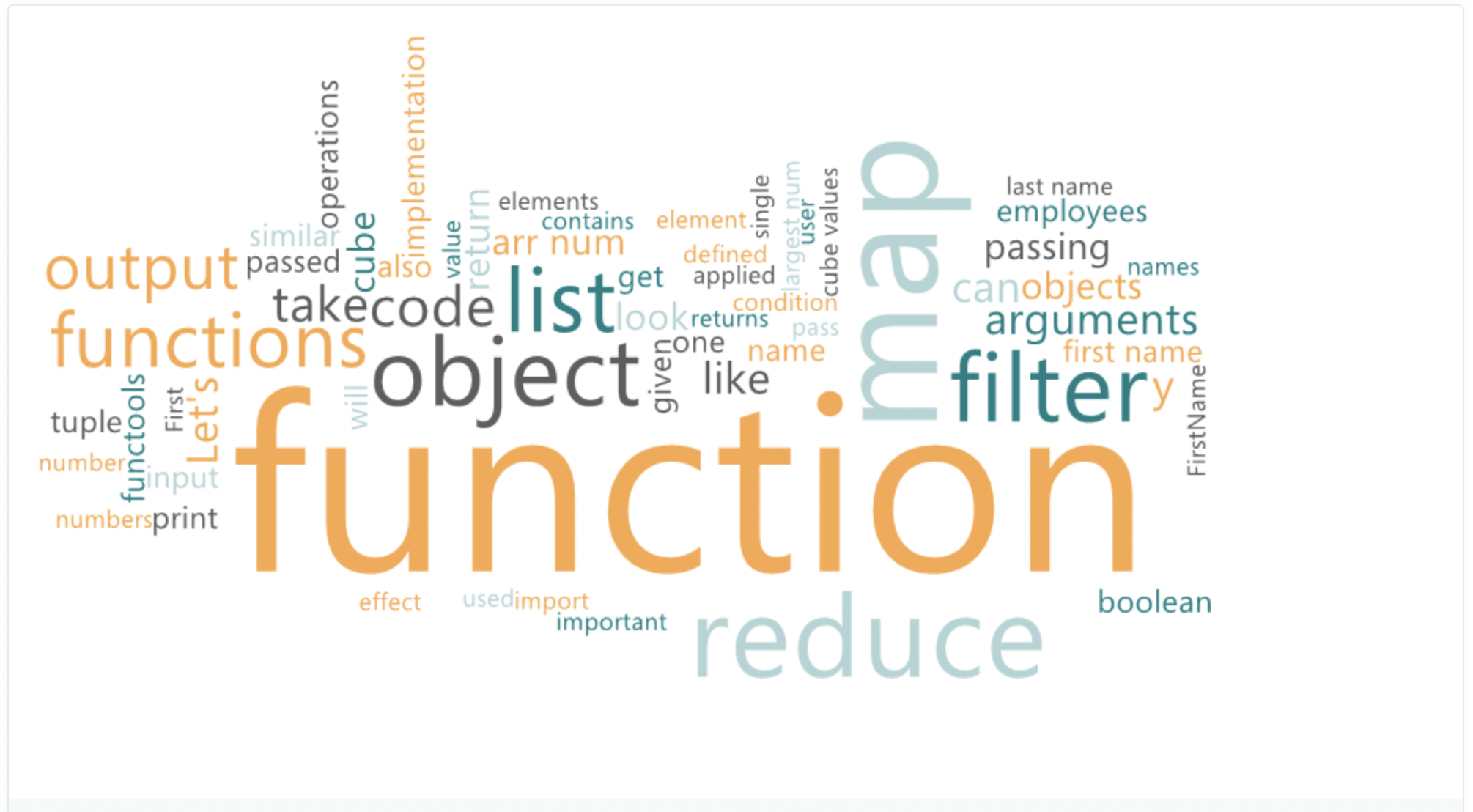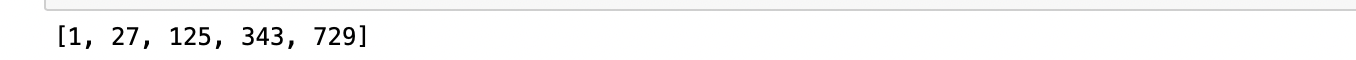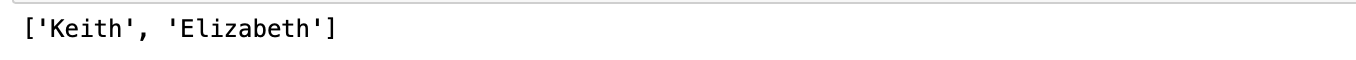Akansha Khandelwal — Published On September 12, 2021 and Last Modified On September 19th, 2022

This article was published as a part of the Data Science Blogathon

Map, Filter, and Reduce are paradigms of functional programming.

## What is functional programming?

Functional programming, as the name suggests, computes through the evaluation of functions. They allow us to write simpler, shorter code with faster implementation methods. In functional programming, code relies entirely on the evaluation of pure functions.Source: Image created by the author

## What are Pure Functions in Functional Programming?

When the output of a function comes directly from the inputs it takes, without any noticeable side-effects, we call it a pure function. We compute by passing functions as arguments, without making changes to the overall program’s state or touching mutable data.

Below we proceed to discuss the nuances of functional programming, let’s look at the key advantages of a code written in with functional programming paradigm:

1. Abstraction: By leveraging functions, we have abstracted our code. Once we have written the function, tested it, and checked its output, we can relax, knowing that whenever we call the function, it will produce the same output for which we defined the function.

1. Transparency: As we write pure functions, we are certain of the behavior of the code in any environment. This takes away the possibility of any side effects and makes debugging easy.

2. Parallel computing: All operations can potentially run in parallel as the operations do not cause any side effects

3. High-level paradigm: By utilizing functions, we describe the outcome we want from the function instead of specifying a step-by-step flow.

## Key functions of Functional Programming

Three key functions that form the heart of the functional programming paradigm are Map, Filter, and Reduce.

The important idea here to note is that you are performing operations by passing functions inside other functions. Moreover, as these are pure functions designed to give one particular output, they reduce the probability of bugs in the overall code that may crop up due to change in variables, user inputs, and unintended consequences.

In the below table, I give a crisp summary of the key characteristics of Map, Reduce, and Filter. Do pay attention to the effect of the function that we pass as an argument and the effect of the input on the output.

Now, let’s look at Map, Reduce, and Filter with examples.

## Map Function

In the map function, the function you pass as an argument will get applied to the iterable object.

If you are familiar with list comprehensions, the Map function has a similar effect. We use it when we need to map or implement functions on various elements at the same time.

The map function takes the following arguments:

`map(function, iterable object)`

Function: Can be a user-defined function or a lambda function. Lambda is an anonymous function that can take multiple arguments. They can have only one expression.

Iterable object: Objects like strings, tuple, list, set, dictionary

Suppose we have a list named FirstName which contains the First Name of all employees, and we have another list that contains LastNames of all employees. The desired output is to display the full name. You could achieve this with for loops, but that would require multiple lines of code and would be tedious. Map functions allow us to achieve this with a single line of code.

Input:

In map function’s implementation, the lambda function which we have defined as lambda x, y:x+” “+y would return a lambda object, but the map handles the job of passing each element of the iterable to the lambda object and storing the value we get in a map object. Using the list function on the map object, you finally get the output list.

Now let’s take an example where we would like to find a cube of all numbers. We could also do it by passing a function as an argument.

Let’s first create a function that returns a cube of an input

```def cube(x):
return x**3```

Now let’s pass this to the map function

```arr_num = [1,3,5,7,9]
cube_values = list(map(cube, arr_num))
print(cube_values)```

Output:## Filter Function

‘Filter’ behaves similar to the map function but also requires the function to look for a condition, which is boolean. The filter then returns elements from the iterable object that satisfy the condition. In essence, it filters away objects that do not satisfy the boolean logic.

Arguments for a filter function

filter(function, iterable object)

Function: The function object passed to the filter function should always return a boolean value, like if the item is an integer or not.

Iterable object: Similar to the map function, it accepts all iterable objects like string, list, tuple, set, dictionary.

Let’s look at how we can use the filter function to filter employees whose firstname ends with ‘h’

```names = list(filter(lambda x: ( x[-1]=='h'),first_name))
print(names)```

We would get below output:## Reduce Function

‘Reduce’ enables pair-wise operations on the iterable object. It uses the result from each operation defined in the passed function, with each successive element. This has the effect of reducing the total items on which pyton will apply the function until only one ultimate value remains.

Before we look at the arguments of reduce function, it’s important to note that reduce has to be imported from the functools library in python

Here’s the command to import

`from functools import reduce`

Arguments:

reduce(function, iterable object)

Function:

• The function passed to the reduce function will take two input values to perform pair-wise operations.

• Leads to single final output.

Iterable objects: string, list, tuple, set, dictionary.

Let’s look at an example to understand the reduce function:

We have a list of numbers, and we would like to find the largest number.

```arr_num = [110,569, 784, 377, 900, 126]
largest_num = reduce(lambda x, y: x if x>y else y, arr_num)
print(largest_num)```

In the code snippet given above, we are importing the functools library to access reduce functions. In implementing the reduce function, the lambda x, y: x if x>y else y, we are returning x if x>y else we return y.

While I have given simple examples to bring out the meaning of map, filter, and reduce functions, they are powerful and can achieve complex tasks elegantly. Since we are achieving our outcome by passing functions, we are assured of the consistency of the output and the predictability of our code. This also makes the code easier to review and change, if required, in the future. No wonder these functional programming tools are indispensable when writing programs you want to be stable over a long period.